# How to Calculate the Volume, Length, Width and Height of a Cuboid | Nickzom Calculator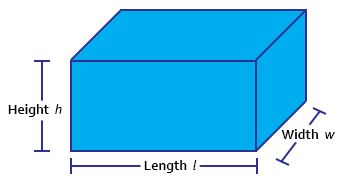The image above represents a cuboid.
To compute the volume of a cuboid requires three essential parameters which are the length, width and height of the cuboid.

The formula for computing the volume of a cuboid is:

V = lwh

Where:
V = Volume of the Cuboid
l = Length of the Cuboid
w = Width of the Cuboid
h = Height of the Cuboid

Let’s solve an example
Find the volume of a cuboid with a length of 6 cm, width of 2 cm and a height of 10 cm.

This implies that:
l = length of the cuboid = 6
w = width of the cuboid = 2
h= = height of the cuboid = 10

V = lwh
V = 6 x 2 x 10
V = 120

Therefore, the volume of the cuboid is 120 cm3.

Calculating the Length of a cuboid when Volume, Width and Height are Given

The formula is l = V / (w)(h)

Where;
V = Volume of the Cuboid
l = Length of the Cuboid
w = Width of the Cuboid
h = Height of the Cuboid

Let’s solve an example:
Find the length of a cuboid with a volume of 440 cm3 , a width of 5 cm and a height of 11 cm.

This implies that;
V = Volume of the cuboid = 440 cm3
w = width of the cuboid = 5 cm
h  = height of the cuboid = 11 cm

l =  V / (w)(h)
l = 440 / (5)(11)
l = 440 / 55
l = 8 cm

Therefore, the length of the cuboid is 8 cm.

Calculating the Width of a cuboid when Volume, Length and Height are Given

The formula is w = V / (l)(h)

Where;
V = Volume of the Cuboid
l = Length of the Cuboid
w = Width of the Cuboid
h = Height of the Cuboid

Let’s solve an example:
Find the width of a cuboid with a volume of  180 cm3 , a length of 6 cm and a height of 10 cm

This implies that;
V = Volume of the cuboid = 180 cm3
l = length of the cuboid = 6 cm
h  = height of the cuboid = 10 cm

w =  V / (l)(h)
w = 180 / (6)(10)
w = 180 / 60
w = 3 cm

Therefore, the width of the cuboid is 3 cm.

Calculating the Height of a cuboid when Volume, Length and Width are Given

The formula is h = V / (l)(w)

Where;
V = Volume of the Cuboid
l = Length of the Cuboid
w = Width of the Cuboid
h = Height of the Cuboid

Let’s solve an example:
Find the height of a cuboid with a volume of 195 cm3 , a length of 5 cm and a width of 3 cm

This implies that;
V = Volume of the cuboid = 195 cm3
l = length of the cuboid = 5 cm
w  = width of the cuboid = 3 cm

h =  V / (l)(w)
h = 195 / (5)(3)
h = 195 / 15
h = 13 cm

Therefore, the height of the cuboid is 13 cm.

Nickzom Calculator – The Calculator Encyclopedia is capable of calculating the volume of a cuboid.

To get the answer and workings of the volume of a cuboid using the Nickzom Calculator – The Calculator Encyclopedia. First, you need to obtain the app.

You can get this app via any of these means:

To get access to the professional version via web, you need to register and subscribe for NGN 1,500 per annum to have utter access to all functionalities.
You can also try the demo version via https://www.nickzom.org/calculator

Once, you have obtained the calculator encyclopedia app, proceed to the Calculator Map, then click on Mensuration under the Mathematics section.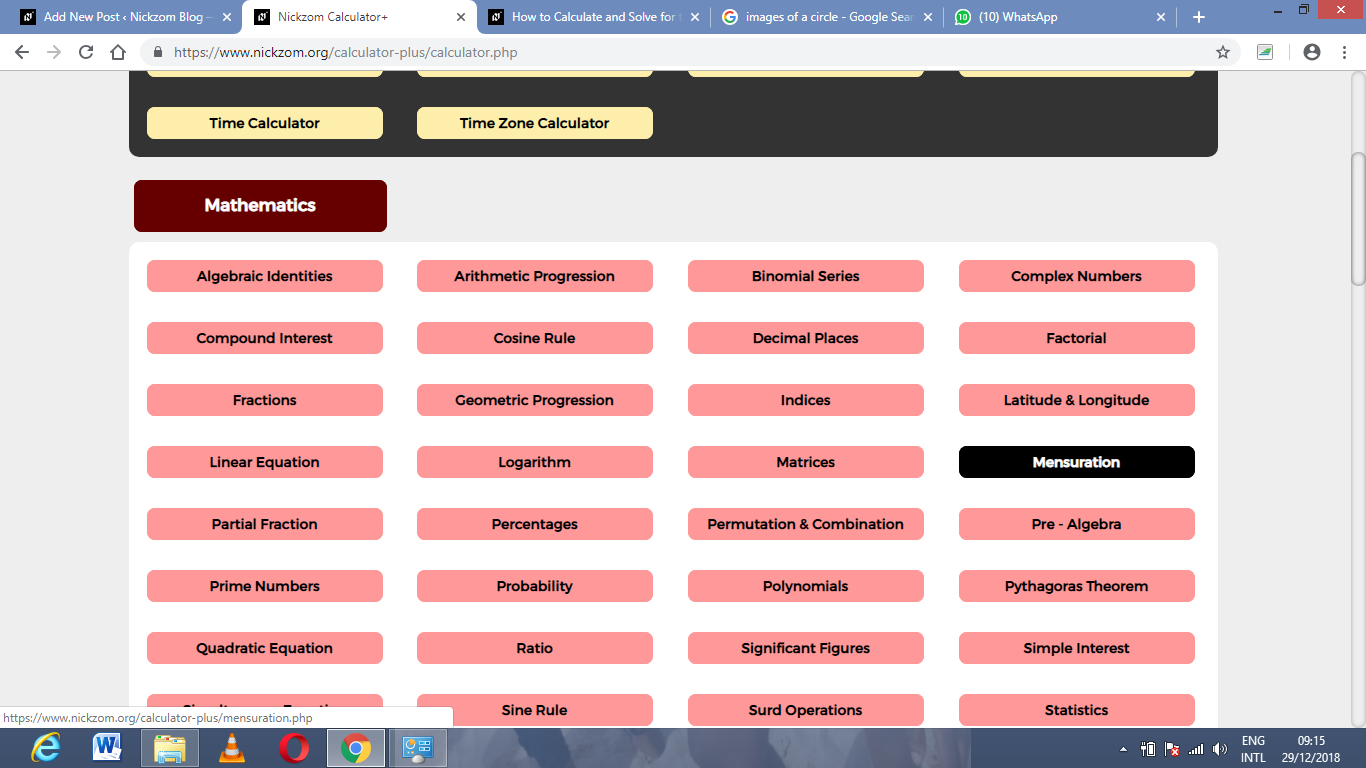Now, click on Volume of a Cuboid under MensurationThe screenshot below displays the page or activity to enter your values, to get the answer for the volume of a cuboid according to the respective parameters which are the width, length and height of the cuboid.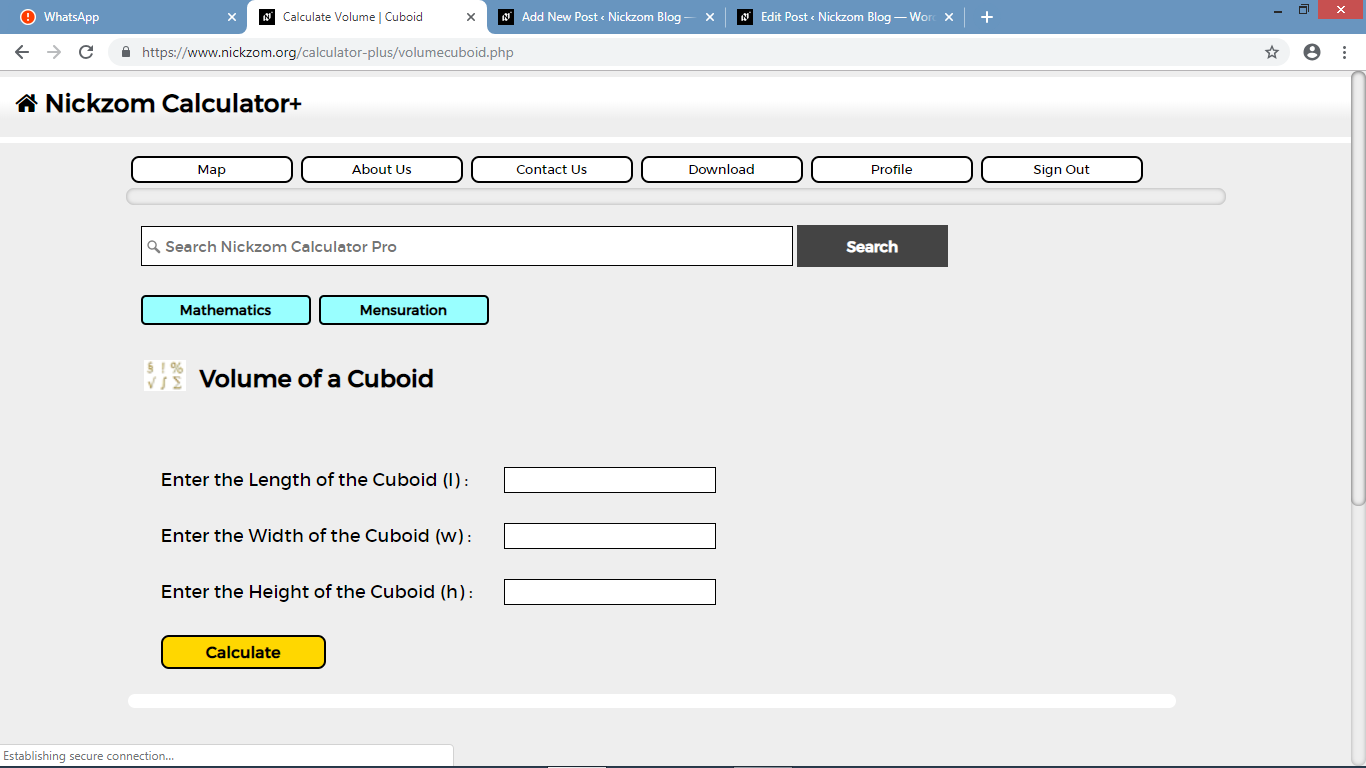Now, enter the values appropriately and accordingly for the parameters as required by the example above where the width of the cuboid is 2 cm, length of the cuboid is 6 cm and the height of the cuboid is 10 cm.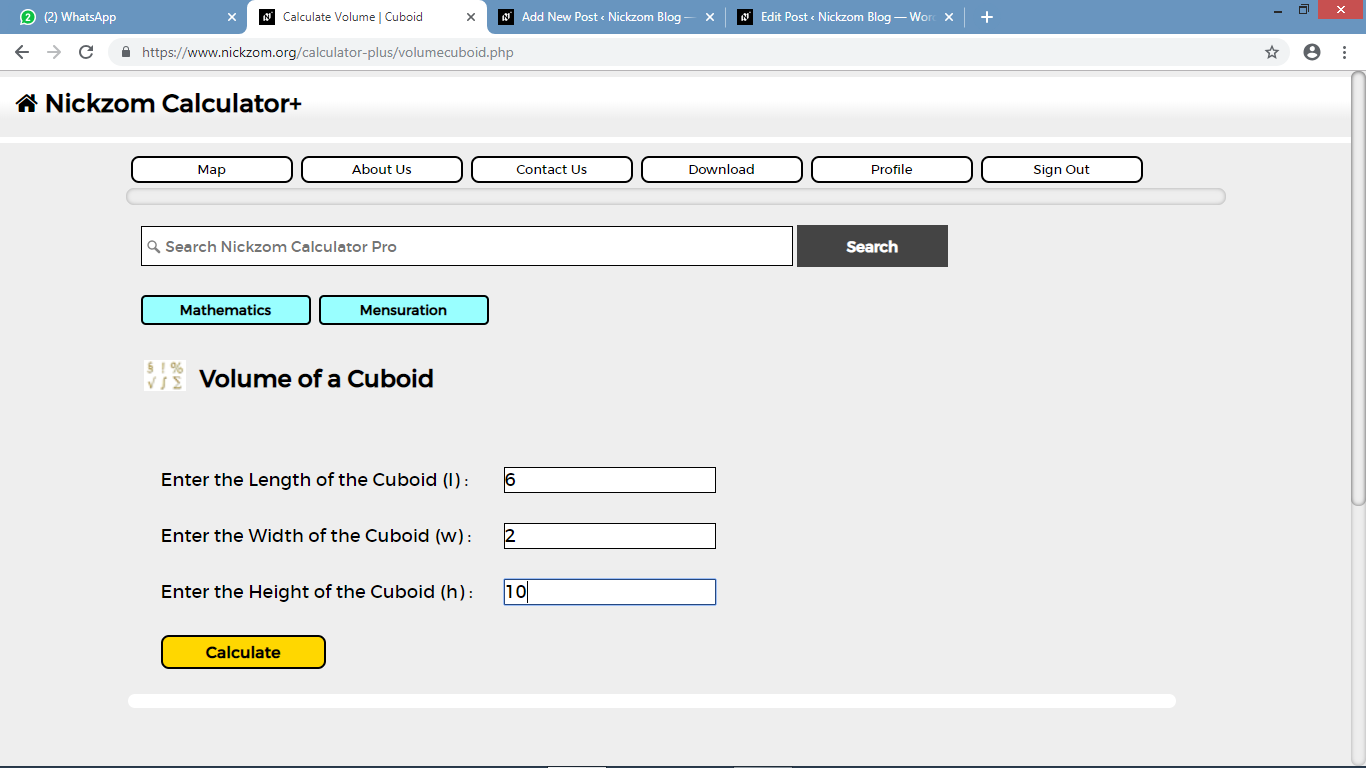Finally, click on the Calculate button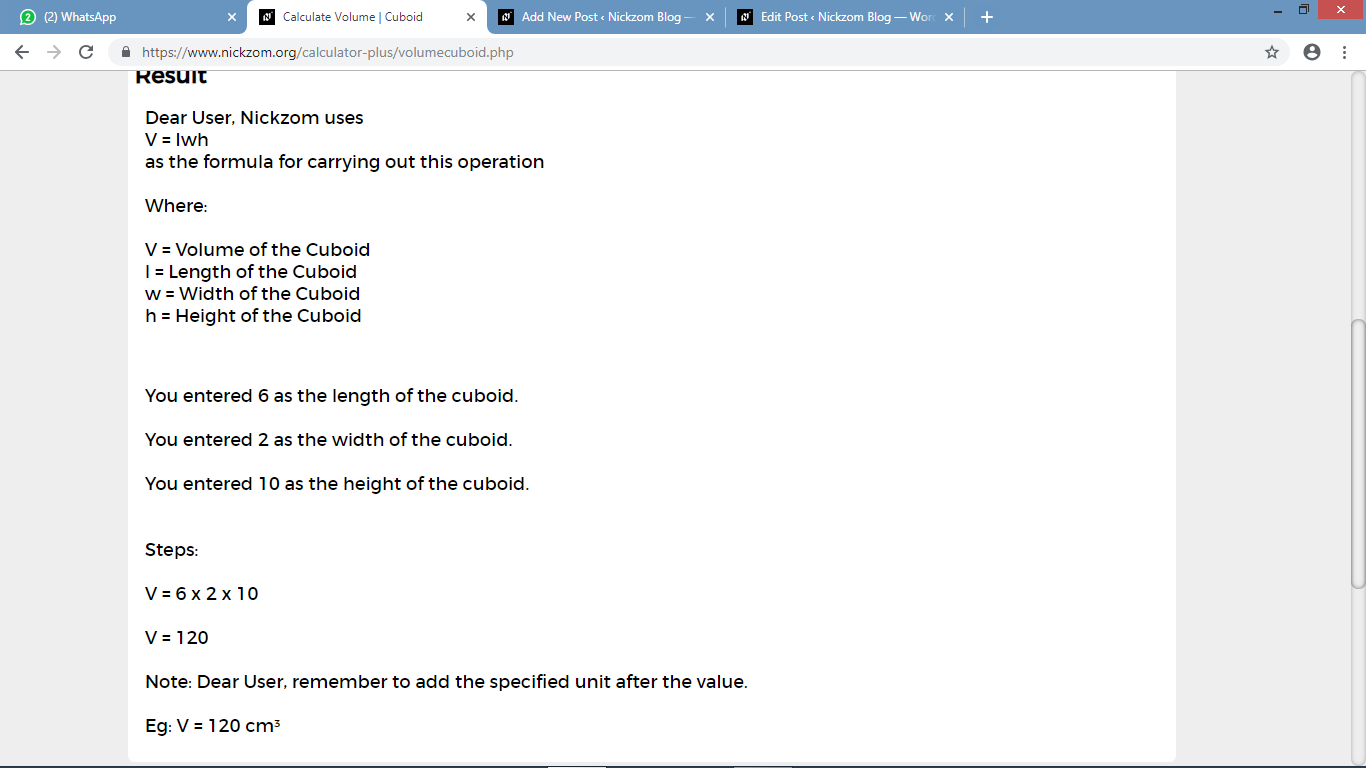As you can see from the screenshot above, Nickzom Calculator – The Calculator Encyclopedia solves for the volume of a cuboid and presents the formula, workings and steps too.